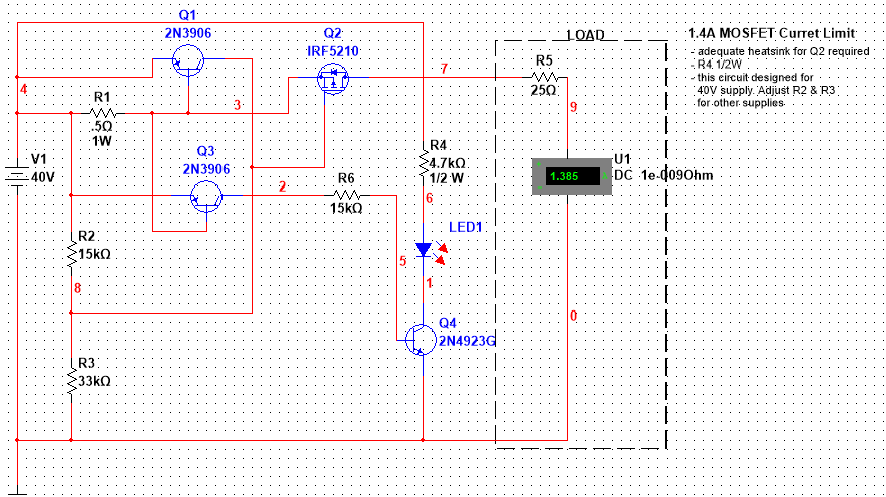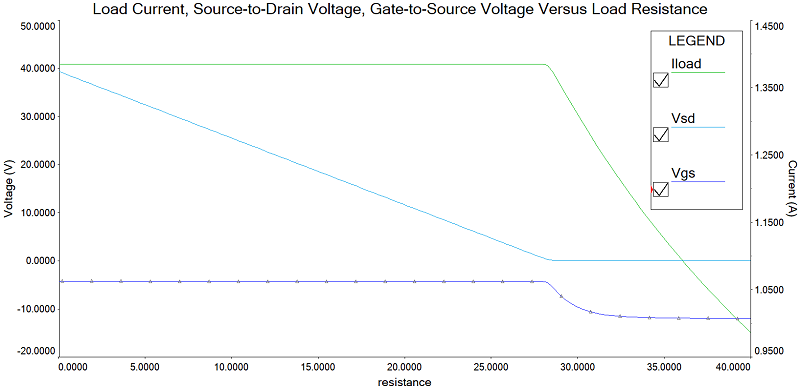## MOSFET Current Limiter### Description

This circuit provides a current-limited DC output voltage. As shown, the circuit limits the output current to a maximum of 1.4 A and includes a visual indicator of a current-limit condition. During normal operation (i.e., load current is less than the limit value) the MOSFET should be fully on. As the load increases (load resistance decreases) to the point at which the current limit is reached, the circuit should decrease the output voltage to ensure the output current design limit is not exceeded.

### Design Details -- How it Works

#### Biasing the MOSFET

Referring to the schematic, R2 and R3 form a voltage divider providing bias to Q2. The supply voltage for this circuit is 40V. From the datasheet for the IRF5210, the minimum gate to source voltage is $V_{GS} = -20V$. Since the gate voltage is supplied from the divider network, we have: $\frac{40R_3}{(R_2+R_3)} - 40 > -20$ Simplifying: $\frac{40R_3}{(R_2+R_3)} > 20 \text{ or } 40R_3 > 20(R_2+R_3)$ Therefore, $$20R_3 > 20R_2 \text{ or } R_2 < R_3$$ However, to turn Q2 on, we must ensure that the gate voltage is less than the gate threshold voltage $V_T$. Moreover, since we want Q2 fully on, we should design for a gate to source voltage $<-10V$. Note that this value can be obtained from the datasheet. Therefore, $$\frac{40R_3}{(R_2+R_3)} -40 < -10 \text{ or } 10R_3 < 30R_2$$ Rearranging yields:$$R_2 > \frac{1}{3}R_3$$ This yields the following range for R2: $$\frac{1}{R_3} < R_2 < R_3$$ Selecting $R_3=33k\Omega$ implies$$11k\Omega < R_2 < 33k\Omega$$. Given this, a value of $R_2=15k\Omega$ was selected. These values for $R_2$ and $R_3$ yield a bias voltage of 27.5V or $$V_{GS} = 27.5 - 40 = -12.5V$$ which is well below the fully-on voltage of -10V and well above the minimum gate to source voltage of -20V.

#### Current Limit

The current limiting capabilities of the circuit are provided by sense resistor $R_1$ in conjunction with the 2N3906 PNP transistor, Q1 . A voltage drop will develop across sense resistor, $R_1$, as current, $I_L$, passes from the positive supply voltage through Q2 to the load. This voltage drop is given by Ohm's law as $I_LR_1$ and appears across the $V_{EB}$ junction of Q1. Transistor, Q1, will remain off until its emitter to base voltage exceeds the emitter to base forward diode drop (approximately .7V). Therefore, from Ohm's law, the value of the current through $R_1$ at which Q1's emitter to base voltage exceeds the .7V diode drop is given by: $.7V = .5I_L$ or $I_L=1.4A$. How does this limit current, $I_L$? As $V_{EB}$ increases above .7V, current begins to flow out of the collector of Q1 and through $R_3$ to ground. Therefore, the total current passing through $R_3$ increases by the amount supplied by the collector of Q1. The net effect of increased current through $R_3$ is (again, by Ohms Law) an increase in the gate voltage of Q2, and therefore an increase in the $V_{GS}$ voltage. $V_{GS}$ will continue to rise until it approaches $V_T$ at which point Q2 will begin to shutdown, reducing the load current $I_L$, and hence the collector current of Q1; thereby, reducing the $V_{GS}$ voltage. Ultimately, a balance will be reached whereby Q2 supplies sufficient current to supply a $V_{GS}$ value that limits $I_L$ to approximately 1.4A.

#### Current Limit Indicator

Transistors Q3 and Q4 in conjunction with LED D2 and limiting resistor R5 form the current limit indicator sub circuit. The behavior of the current limit indicator sub circuit is similar to the current limit circuit described above in that the collector of Q3 provides current to the base of Q4 in response to an increase in the $V_{EB}$ voltage of Q3 as the current through sense resistor R1 increases. Note that a 2N4923 transistor was chosen for Q4 rather than a 2N3904 because of the collector to emitter voltage.